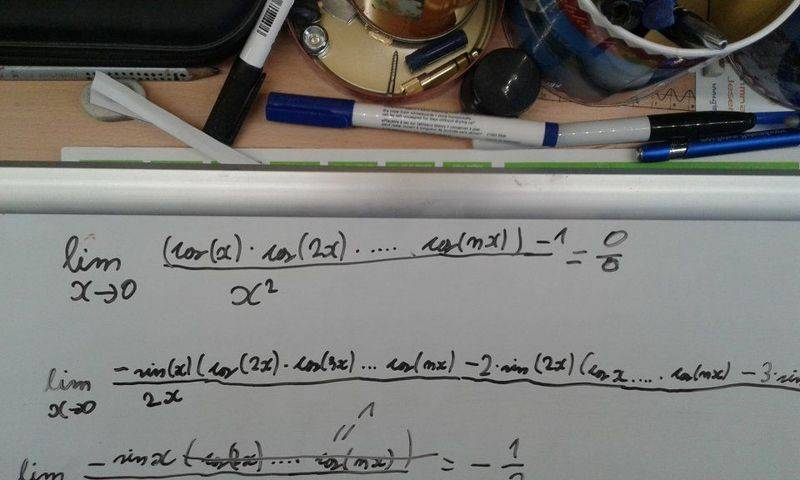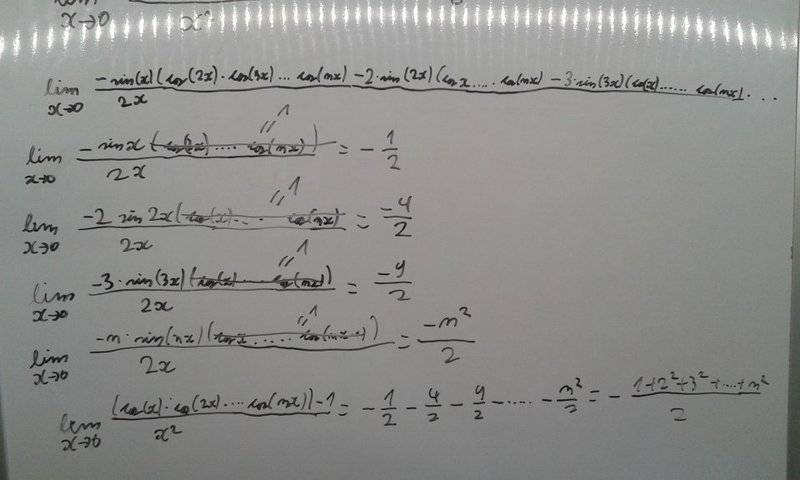# Limit problem ( can't find the mistake)

Matejxx1

## Homework Statement

Hi. My professor asked me if I know to solve this limit and I tried doing it, however I didn't get the same answer as him.
Question: What is the limit of (cos(x)*cos(2x)*cos(3x)*...*cos(nx)-1)/x^2 as x approches 0/

## The Attempt at a Solution[/B]
So to find this limit I used L'Hospital's rule.
I derived the top and bottom function and I noticed that each part of the derivative can be calculated using sin(x)/x as x ->0 =1. Then I noticed that the whole thing equals -(1^2+2^2+3^2+.....n^2)/2
However my professor said that the whole thing should equal -n(n+1)(2n+1)/2

Homework Helper

## Homework Statement

Hi. My professor asked me if I know to solve this limit and I tried doing it, however I didn't get the same answer as him.
Question: What is the limit of (cos(x)*cos(2x)*cos(3x)*...*cos(nx)-1)/x^2 as x approches 0
View attachment 101263

/

## The Attempt at a Solution

View attachment 101262 [/B]
So to find this limit I used L'Hospital's rule.
I derived the top and bottom function and I noticed that each part of the derivative can be calculated using sin(x)/x as x ->0 =1. Then I noticed that the whole thing equals -(1^2+2^2+3^2+.....n^2)/2
However my professor said that the whole thing should equal -n(n+1)(2n+1)/2
Your result is correct but not complete. The sum of the squares of the first n integer is n(n+1)(2n+1)/6
http://www.trans4mind.com/personal_development/mathematics/series/sumNaturalSquares.htm

•Matejxx1
Matejxx1
Thank you. So the final answer -n(n+1)(2n+1)/12 would be correct?

•